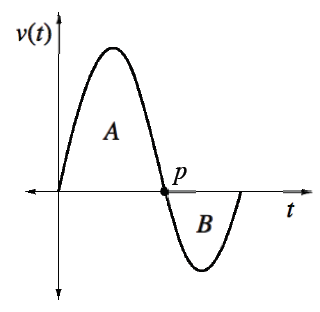### Home > APCALC > Chapter 3 > Lesson 3.2.2 > Problem3-63

3-63.

Answer the following questions using the graph at right, which shows the velocity of a runner over time. The letters $A$ and $B$ represent the areas of the two regions in the diagram.

1. If the runner was at the starting line at $t = 0$, describe her direction during the interval illustrated on the graph at right.

Include information about the direction that the runner was moving.

2. What is the significance of point $p$?

How does the runner's motion change when s/he reaches point $p$?

3. What does region $A$ represent in this situation? That is, what does it tell you about the runner? What about region $B$?

What does area under a velocity curve represent?

4. If $A = 30$ meters and $B = −5$ meters, what does $A + B$ represent in this situation? Why is $B$ negative?

Compare and contrast 'displacement' with 'total distance.'